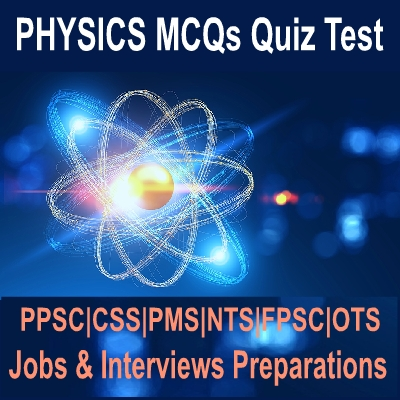Friday, 10 January 2020

# Physics Solved NTS Tests MCQs

Hello Students Today i am sharing with you important Physics Question Answers Solved MCQs. All of These MCQs are helpful for Preparations of National Testing Service - NTS Jobs Tests preparations of Physics. All of these Physics Competitive Exams Multiple Choice Questions with solutions are easy to learn with solved answer of each question.Solved important MCQs of physics test

Many of the Subject type tests are taken by the NTS just like Engineering jobs tests, Physics Teachers, MCAT, ECAT and others similar tests in which Physics Question answers are asked. These solved Objective Type Nts Physics MCQs will helpful for Preparations of tests and interviews of Physics.

### Solved Physics Objective Type Quiz MCQs Preparations With Answers

Q.1: The number of significant figures in the length of a bar 6200mm measured by meter rod is--------- ?
1. 4
2. 3
3. 2
4. None of these
A
Q.2: The direction of the linear velocity of a body moving in a circle is--------- ?
1. along the axis of rotation
2. along the tangent
3. directed towards the centre
4. directed away from the centre
D
Q.3: In case of planets the necessary acceleration is provided by-------- ?
1. Gravitational force
2. Frictional force
3. Coulomb force
4. Centripetal force
A
Q.4: The shaft of a motor rotates at a constant angular speed of 360rev/min. Angle turned through in 1 sec in radian is------ ?
1. π
2. 12π
D
Q.5: A satellite moving around the earth constitutes------ ?
1. An inertial frame of reference
2. Non-inertial frame
3. Neither inertial nor non-inertial
4. Both inertial and non-inertial
B
Q.6: Terminal velocity is--------- ?
1. Uniform
2. Maximum
3. Uniform and maximum
4. Neither uniform nor maximum
C
Q.7: Speed of efflux can be determined by applying------ ?
1. Bernoulli’s theorem
2. Torricelli’s theorem
3. Venture relation
4. All
B
Q.8: Venturi meter is used to measure--------- ?
1. Fluid pressure
2. Fluid density
3. Fluid speed
4. None
A
Q.9: A chimney works best if air exposed to the chimney is------ ?
1. Stationary
2. Moving
3. Moving slowly
4. Moving fast
D
Q.10: The profile of aeroplane wing which lifts it up is called as------- ?
1. Wing shape
2. Aerofoil profile
3. Curved profile
4. None of these
B
Q.11: In S.H.M. the velocity of a particle is maximum at--------- ?
1. mean position
2. extreme position
3. middle between the mean and extreme position on the right side
4. middle between the mean and extreme position on the left side
A
Q.12: The force responsible for the vibratory motion of the simple pendulum is----------- ?
1. mg cosθ
2. mg sinθ
3. mg tonθ
4. mg
B
Q.13: The displacement of SHM is written as X = X`sinθt if the displacement is written by X = Xθ sont then phase constant will be equal to--------- ?
1. 45°
2. 90°
3. 180°
C
Q.14: The sharpness of resonance is------ ?
1. Directly proportional to the damping force
2. Inversely proportional to the damping force
3. Equal to the square of damping force
4. Equal to the square of damping force
B
Q.15: Displacement of the body in S.H.M is equal to amplitude when the body is at-------- ?
1. Mean position
2. Elsewhere
3. Extreme position
4. None
C
Q.16: Which one of the following radiations has the strongest photon ?
1. T.V waves
2. Microwaves
3. X-rays
4. γ-rays
D
Q.17: Stopping potential for a metal surface in case of photoelectric emission depends on-------- ?
1. the threshold frequency for the metal surface
2. the intensity of incident light
3. the frequency of incident light and work function of the metal surface
4. all of the above
C
Q.18: Davison Germer experiment indicates--------- ?
1. Interference
2. Polarization
3. Electron diffraction
4. Refraction
C
Q.19: A three-dimensional image is obtained by-------- ?
1. Electron microscope
2. Scanning electron microscope
3. Magnetic imaging
4. None of the above
B
Q.20: Unit of power of a lens is---------- ?
1. meter
2. watt
3. diopter
4. horsepower
C
Share: